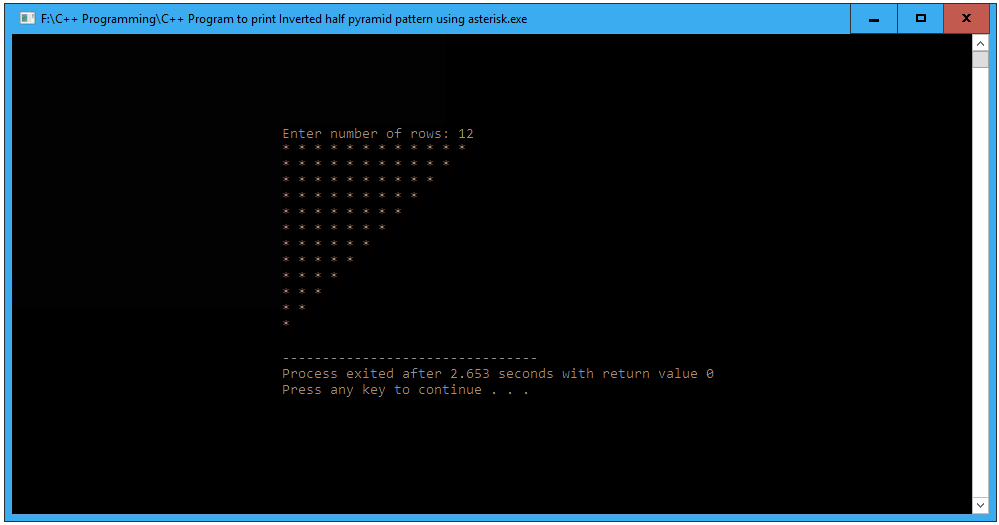# C++ Program to print Inverted half pyramid pattern using * (asterisk)

In this article we will write a C++ Program to print Inverted half pyramid pattern using asterisk. This Program first takes the numbers of rows and uses nested for loops to print Inverted half pyramid pattern.

## C++ Program to print Inverted half pyramid pattern using asterisk

```//C++ Program to print Inverted half pyramid using * (asterisk) pattern

#include <iostream>
using namespace std;

int main()
{
int i, j, row;

cout << "Enter number of rows: ";
cin >> row;

// outer loop is responsible for rows
for(i = row; i >= 1; i--)
{

//inner loop is responsible for columns
for(j = 1; j <= i; j++)
{
cout << "* ";
}

// give line breaks after ending every row
cout << "\n";
}

return 0;
}
```

### When the above C++ program is compile and run, this will produce the following result: